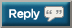# Thread: How do I figure out how much many pounds equals 1% of fat?

1. ## How do I figure out how much many pounds equals 1% of fat?

How do I figure out how much many pounds equals 1% of fat? I am 5'8, male, 187 lbs . at 25% bodyfat.2. If you had paid attention in algebra you would be able to do this...

You need to first figure out how pounds of fat you have. Then you can divide the pounds of fat you have by the percent bodyfat because that will get you pounds of fat per percent bodyfat.3. How do I figure out how much many pounds equals 1% of fat? I am 5'8, male, 187 lbs . at 25% bodyfat.
Your fat weight is 187*.25 = 46.75lbs

1% of your fat weight is 46.75*.01 = .4675 lbs4. If you had paid attention in algebra you would be able to do this...

You need to first figure out how pounds of fat you have. Then you can divide the pounds of fat you have by the percent bodyfat because that will get you pounds of fat per percent bodyfat.
Doing that would just give you 1% of your body weight.5. Ok, contrary to what Saturnal said (as I have to disagree with him based on what I think you are saying) if you are a 187lb man, with 25% bodyfat, to drop that by 1% (I think this is what you mean) you need to drop 1.87lbs of fat (1% of your weight). Of course, this is an approximation, because if you drop 1.87lbs, you will only be 186.13lbs, and you would now have only 44.88lbs of fat which is actually 24.1% bodyfat because of weight loss so it is not exactly right...6. Ok, contrary to what Saturnal said (as I have to disagree with him based on what I think you are saying) if you are a 187lb man, with 25% bodyfat, to drop that by 1% (I think this is what you mean) you need to drop 1.87lbs of fat (1% of your weight). Of course, this is an approximation, because if you drop 1.87lbs, you will only be 186.13lbs, and you would now have only 44.88lbs of fat which is actually 24.1% bodyfat because of weight loss so it is not exactly right...
If he wanted to go from 25% bf to 24%, then he'd have to lose 2.46lbs of fat.

But that number changes as the bf gets lower, so it's hard to tell what he's asking for exactly. Yeah, the bf would drop 1% the first week if he lost 2.46lbs, but if he lost that amount next week, the bf would drop by more than 1%.7. Me and lordofchaos are on the same road. Saturnal is on another. They both happen to be right.

What we need to find out is what exactly the guy wants. The question wasn't clear enough for us to be able to tell.8. If you wanna find out how much 1% of your fat weight is, then see my first reply. If you want to figure out how much fat you'd have to lose to reduce your body fat percentage by 1%, then this is how you'd do that:

New fat weight = (New body fat % x Lean weight)/(1 - new body fat %)

In the original example, new body fat % would be .24, and lean weight would be 140.25. So the new fat weight would be 44.29.

Then just subtract your new fat weight from your old fat weight, and that would tell you how much fat you'd have to lose in order to reduce your body fat % by 1%.

In the same example, (old fat weight) minus (new fat weight) would be 2.46 lbs.9. If you wanna find out how much 1% of your fat weight is, then see my first reply. If you want to figure out how much fat you'd have to lose to reduce your body fat percentage by 1%, then this is how you'd do that:

New fat weight = (New body fat % x Lean weight)/(1 - new body fat %)

In the original example, new body fat % would be .24, and lean weight would be 140.25. So the new fat weight would be 44.29.

Then just subtract your new fat weight from your old fat weight, and that would tell you how much fat you'd have to lose in order to reduce your body fat % by 1%.

In the same example, (old fat weight) minus (new fat weight) would be 2.46 lbs.

You are completly right man. That is the correct math (since I did pay attention in all of my math classes) and is the only way to calculate your body fat weight.10. Sorry to open a post from the past but seems to be the answer is in front of my face and I just can't quite work it out. So I'm 186lb with about 21% bf - my end goal would be to get around 15% bf - is there a target amount of weight i'll need to lose to drop that amount and if so can you advise the calculation to work this out11. 186 lbs @ 21% bf = 39 lbs fat/147 lbs lbm.

Assuming zero muscle loss you'll ned to cut down to ~173 lbs to be 15% bf. (173 - 147)/173 = 15#### Posting Permissions

• You may not post new threads
• You may not post replies
• You may not post attachments
• You may not edit your posts